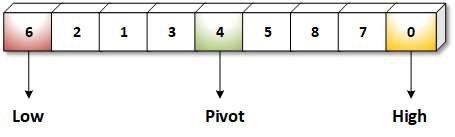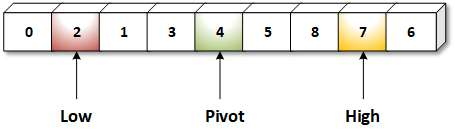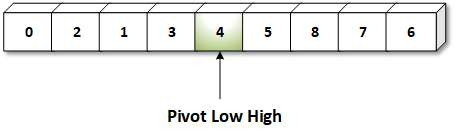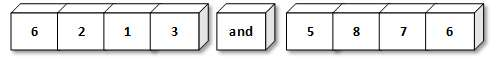# Array C Programing

Quick sort uses the idea of Divide and Conquer technique. This algorithm is used in order to sort an array in ascending order, finds the element that divides the array into two halves in such a way that the element in the left sub array are less than and elements in the right sub array are greater than the dividing element.

Then these two sub arrays are sorted separately This procedure is recursive based on the criteria that the number of elements in the sub array are net more than one.

Algorithm:

Q-Sort (array, first, last)

where, Array represents list ofelements

First represents the position of first element in the list.
Last represents position of last element in the list.

Step 1: [initially]

low = first
high = last
pivot = array [(low + high)/2]
[middle element ofthe list]

Step 2: Repeat through step 7 while (low = high)

Step 3: Repeat through step 4 while (array [low]<pivot)

Step 4: Low = low + 1

Step 5: Repeat step 6 while (array [high] >pivot)

Step 6: High = high - I

Step 7: If(low <high)

(i). temp = array [low]
(ii). array [low] = array [high]
(iii). array [high] = temp
(iv). low = low + 1
(v). high = high - 1

Step 8: If(first < high)

Q-sort (array, first, last)

Step 9: If(low < last)

Q-sort (array, low. last)

Step 10: Exit

Example:low = first = I
high last = 9
Pivot = array [(I + 9)/2] = array  = 4

while (low < high)
{
while (array (low) < pivot)
low ++
while (array [high] > pivot)
high --;
if(low =< high)

{

temp = array [low]

array [low] = array [high]

array [high] = temp

low = low + 1

high = high + 1

}

}

So new list isNow, low = 2, high = 8

Repeat while loop

and new list is2 new sublists will beContinue above with each sublists and finally we
get a sorted list.